# Circuit analysis consisting of an ideal diode.

• Engineering
• ShreyasR
Case (ii) If D2 is assumed to be reverse biased (replaced by an open circuit): We have an open circuited branch in parallel with the 10 kΩ resistance. Hence, current flows through the two 10 kΩ resistors. (effectively in series) By applying voltage divider rule, we obtain Vo = 15(10k/20k) = 7.5 V. The anode voltage of the diode VA = Vo = 7.5 V. The cathode voltage of the diode Vk = 10

## Homework Statement

Find the voltage Vo for the following circuit. Consider the diodes to be ideal.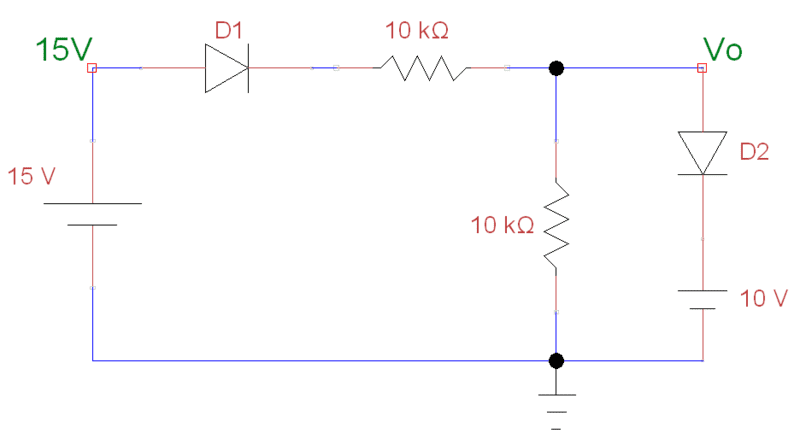## Homework Equations

When a diode is forward biased, Diode resistance = 0Ω (Short circuited)
When a diode is reverse biased, Diode resistance is infinite (Open circuit)

Ohm's Law: V = IR

Voltage divider rule.

Basic Nodal analysis.

Kirchoff's voltage law.

## The Attempt at a Solution

1) Undoubtedly, the diode D1 is forward biased. Hence it is replaced by a short circuit.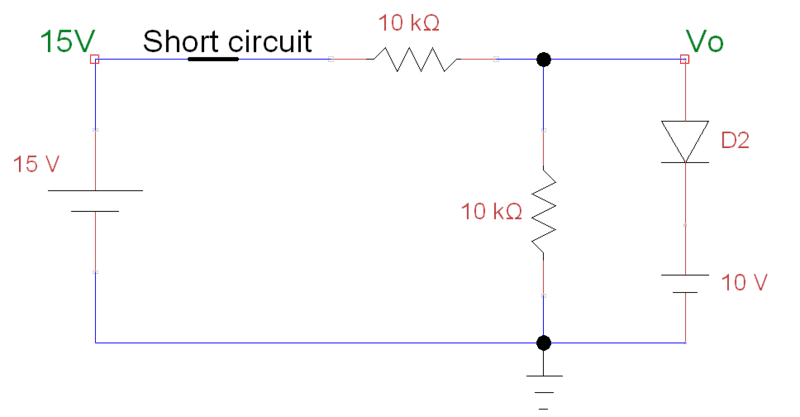2) Now there are two possibilities for D2.
Case (i) If D2 is assumed to be forward biased (replaced by a short circuit):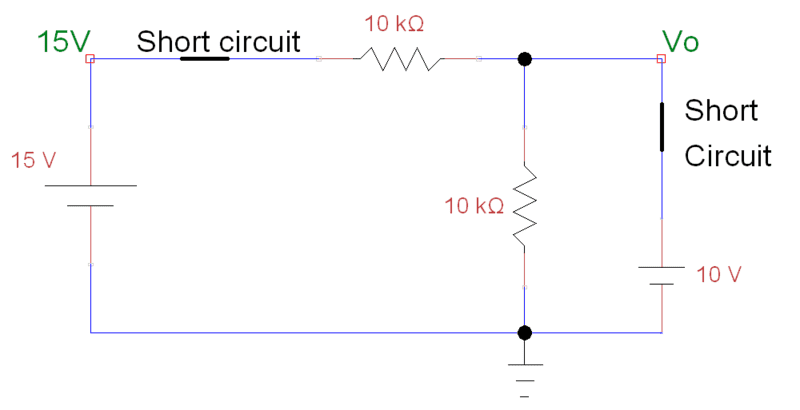We directly obtain Vo = 10 V
Current flows in the branch containing D2. (short circuit path)

By applying Kirchoff's voltage law, we get
15V - I*(10 kΩ) -10V = 0

I = 0.5 mA
We infer that 0.5 mA flows through diode D2 from the P side to N side (Anode to cathode), without any potential drop across the diode. Hence, the assumption that the diode D2 is forward biased is CORRECT.

Case (ii) If D2 is assumed to be reverse biased (replaced by an open circuit):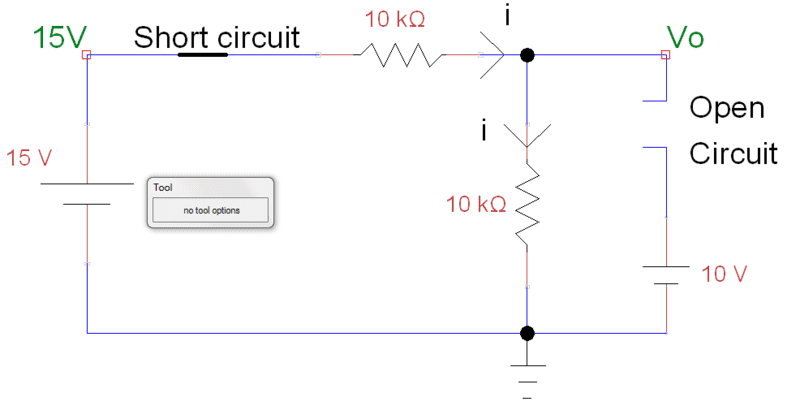We have an open circuited branch in parallel with the 10 kΩ resistance.
Hence, current flows through the two 10 kΩ resistors. (effectively in series)

By applying voltage divider rule, we obtain
Vo = 15(10k/20k) = 7.5 V.

The anode voltage of the diode VA = Vo = 7.5 V.
The cathode voltage of the diode Vk = 10 V.
Hence, VA < Vk ...

As VA < Vk, and no current is flowing though the diode, the assumption that the diode D2 is reverse biased is CORRECT.

These are the two different solutions that I have obtained for the same problem, and both seem to be valid, and are not violating any laws. But getting two solutions of voltages for a well defined circuit is not possible isn't it? Where have I gone wrong?

In analyzing D2, start with the voltages on each side of the diode assuming it is OFF first. This is the safest assumption if you are not sure, and it gives you the correct assumption in this case. If you assume it is OFF and analyze the voltages, and it turns out that Va > Vk, then you change your assumption to the diode being ON. Don't start assuming that the diode is ON, because you get something non-physical in this case.

•1 person
ShreyasR said:

## The Attempt at a Solution

2) Now there are two possibilities for D2.
Case (i) If D2 is assumed to be forward biased (replaced by a short circuit):

View attachment 61234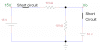We directly obtain Vo = 10 V
Current flows in the branch containing D2. (short circuit path)

By applying Kirchoff's voltage law, we get
15V - I*(10 kΩ) -10V = 0

I = 0.5 mA
We infer that 0.5 mA flows through diode D2 from the P side to N side (Anode to cathode), without any potential drop across the diode. Hence, the assumption that the diode D2 is forward biased is CORRECT.

Where have I gone wrong?

When I went through school it was traditional to show on the diagram what directions were assumed for current.
For the sake of consistency, when writing KCL we noted current entering a node as positive and current leaving a node as negative.

I don't see currents or directions on your sketches.

Where have I gone wrong?

Right Here:
You wrote KVL around outside loop only and of course it holds true.
You failed to finish the problem by applying KCL to the node Vo.
By applying Kirchoff's voltage law, we get
15V - I*(10 kΩ) -10V = 0

I = 0.5 mA
We infer that 0.5 mA flows through diode D2 from the P side to N side (Anode to cathode), without any potential drop across the diode.

Looking at node Vo I see instead 0.5 ma entering it from left and 1ma leaving straight down.
0.5 ma entering and 1 ma leaving does not satisfy KCL, so there must be some current also entering from the right (where your D2 is).
Kirchoff's current law requires the additional 0.5 ma to enter from right, which is backward current flow through D2 which is impossible.
Recall that a diode allows conventional current flow only in direction of the arrow
So the assumption that D2 is forward biased leads to an impossible conclusion.
Therefore that assumption is wrong.

Be more rigorous in setting up your circuit equations.
Haste makes waste my friend.

Last edited:
•1 person
jim hardy said:
Looking at node Vo I see instead 0.5 ma entering it from left and 1ma leaving straight down.
0.5 ma entering and 1 ma leaving does not satisfy KCL, so there must be some current also entering from the right (where your D2 is).

Be more rigorous in setting up your circuit equations.
Haste makes waste my friend.

Silly me! Well thank you! The actual question was a clipper circuit which had an AC source Vin in place of the of the 15 V battery. The main doubt here was what happens in the interval 10 V < Vin < 20 V...

My solution was D1 is forward biased, D2 is reverse biased, And
Vo = Vin/2.

So I was right wasn't I?

The main doubt here was what happens in the interval 10 V < Vin < 20 V...

My solution was D1 is forward biased, D2 is reverse biased, And
Vo = Vin/2.

So I was right wasn't I?

I'd say so.

In my day we had to show our work
Surely your algebra is fresh than mine...
Were this my homework i'd use KVL to derive for voltage across D2 , VD2 = f(Vin)
then express that as an inequality VD2>0
clearly that's when Vin > 20.

Thanks for replying , I was happy to see that you 'got it' . Looks like you had it right.

I had to force myself to be rigorous - when I took mental shortcuts I missed a step or swapped a sign 90% of the time. (You cannot imagine the difficulty that caused me learning FortranII with those nitpicky format statements..)

But with enough drill one gets to where a lot of things become intuitive. You'll know when you're there. I still work most problems two or three times by different approaches, only when they agree am I confident. I did yours as shown and cross-checked that by voltage divider action with D2 open.

I think this is my first participation in homework forum. Thanks for the introduction to it. I hope I followed the rules...

old jim

Last edited:
•1 person
jim hardy said:
I'd say so.

In my day we had to show our work
Surely your algebra is fresh than mine...
Were this my homework i'd use KVL to derive for voltage across D2 , VD2 = f(Vin)
then express that as an inequality VD2>0
clearly that's when Vin > 20.

Thanks for replying , I was happy to see that you 'got it' . Looks like you had it right.

I had to force myself to be rigorous - when I took mental shortcuts I missed a step or swapped a sign 90% of the time. (You cannot imagine the difficulty that caused me learning FortranII with those nitpicky format statements..)

But with enough drill one gets to where a lot of things become intuitive. You'll know when you're there. I still work most problems two or three times by different approaches, only when they agree am I confident. I did yours as shown and cross-checked that by voltage divider action with D2 open.

I think this is my first participation in homework forum. Thanks for the introduction to it. I hope I followed the rules...

old jim

Actually I posted this in Homework forum since it was a numerical problem. This was a question in my exam. I solved it correctly without much of solid reasoning... Now i understand why showing of work and reasoning is important while solving a problem... I thought i had worked enough problems and i solved this by intuition... But when I checked the other (incorrect) solution, I couldn't find the mistake! So rigorous methods are surely essential...!

Thanks a lot!So rigorous methods are surely essential...!

I can't speak for him, but i'll wager Sophiecentaur is smiling.jim hardy said:
I can't speak for him, but i'll wager Sophiecentaur is smiling.Sorry. I guess I did not understand what you meant by that!?

Sorry -
Sophie is a frequent contributor here. His posts are erudite and precise. He advocates rigorous application of proper math to every problem. Look for his posts in engineering forums, they are quite educational.

jim hardy said:
Sorry -
Sophie is a frequent contributor here. His posts are erudite and precise. He advocates rigorous application of proper math to every problem. Look for his posts in engineering forums, they are quite educational.

No, the post doesn't have any mistakes in case you are wondering. Sophie is a guy.